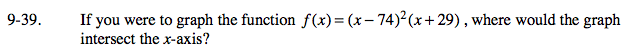Home > A2C > Chapter 9 > Lesson 9.1.2 > Problem9-39

9-39.

If you were to graph the function f (x) = (x − 74)2(x + 29), where would the graph intersect the x-axis? Homework Help ✎Remember that wherever the graph intersects the x-axis, f(x) will be zero. Use the Zero Product Property.

The graph intersects the x-axis at (74, 0) and (−29, 0).